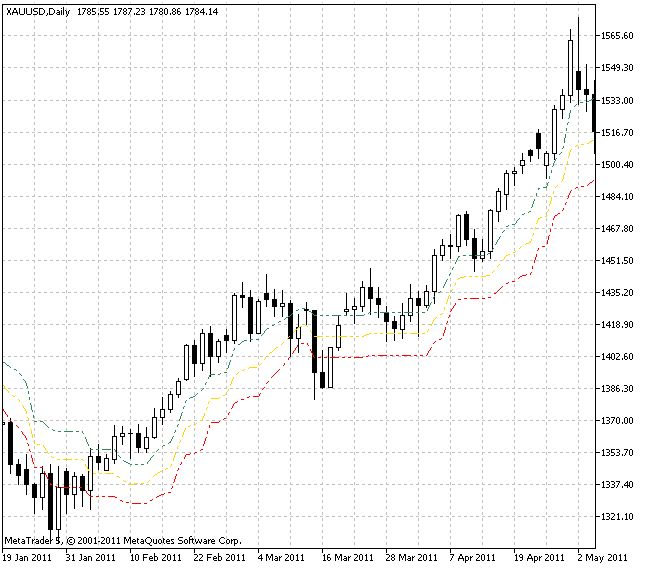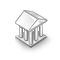• Get access

Interesting script?
So post a link to it -
let others appraise it

You liked the script? Try it in the MetaTrader 5 terminal# Three Tirone Levels - indicator for MetaTrader 5

Views:
6268
Rating:
Published:
2011.09.27 13:08
Updated:
2016.11.22 07:32

Real author:

John Tirone

Tirone Levels are a set of the appropriate support and resistance levels based on a trading range for a certain period of time.

Usually they are used only to improve visual perception of the market price moves. In this case, the Midpoint method is used for the calculation of all three Tirone Levels.

Market minimum and maximum for a certain period of time are calculated at first.

1. To calculate the upper line the minimum is deducted from the maximum, gained value is divided by 3 and the result is deducted from the maximum.
2. To calculate the center line the minimum is deducted from the maximum, this value is divided by 2 and the result is added to the minimum.
3. The lower line is calculated by deducting the minimum from the maximum, dividing the result by 3 and adding the result to the minimum.

The levels are calculated the following way:

Tirone Level 1 = Hhigh - (Hhigh-Llow)/3
Tirone Level 2 = Llow + (Hhigh-Llow)/2
Tirone Level 3 = Llow + (Hhigh-Llow)/3

where:

• Hhigh (Highest High) - the highest price for a certain period, for example, 20 bars.
• Llow (Lowest Low) - the lowest price for a certain period, for example, 20 bars.

Application of Tirone levels resembles the application of Fibonacci correction levels - we should buy when the price crosses Tirone level downwards and sell when the price crosses the level upwards.

Both types of levels are interpreted in a similar way: both methods of graphical analysis use a certain per cent value between a maximum and a minimum to generate the lines and 50% as a probable correction level. Tirone Levels also resemble Quadrant Lines.

Like many other technical analysis methods, Tirone Levels have their followers being one of the simplest trading signals system based on support and resistance, though a considerable amount of traders who work with the intervals less than a day claim that they are less accurate in comparison with other similar methods.Translated from Russian by MetaQuotes Software Corp.
Original code: https://www.mql5.com/ru/code/457MyComment - new comments added without deleting existing ones.3_Level_ZZ_SemaforFive Tirone LevelsVariation Index